# Tensorflow学习笔记（一） 基础

Posted by SkyHigh on October 10, 2016

# Tensorflow学习笔记（一） 基础

## 0.DeepLearning编程框架

• 用于参数配置：
caffe提供了很便捷的神经网络搭建和命令行工具，加之model zoo里面大量预训练好的模型(主要是图像相关的)可以做fine-tuning，因此使用在图像相关的研究和应用上非常方便。
• 用于编程实现：
Theano以及搭建于其之上的Keras和Lasagne似乎颇受research派系同学的偏爱，自动求导是它的优势之一。
MXnet对显存的利用率高，并且支持C++, Python, Julia, Matlab, JavaScript, Go, R, Scala这么多种语言，编写起来也比较简易。

## 1.Tensorflow的名词解释

• 所有的输入、参数输出都以张量的形式流动和传动；
• 一旦计算图（Computation Graph）绘制好，在运行图时就能够自动求导（使用BP的思路，就是链式法则反向传导误差），也就是说，你只需要写好损失函数，之后交给Tensorflow来处理就好了。

• 使用张量(tensor)表示数据。
• 使用图(graph)来表示计算任务。
• 在被称之为会话(Session)的上下文(context)中执行图。
• 通过变量 (Variable)维护状态。
• 使用feed和fetch可以为任意的操作(arbitrary operation)赋值或者从其中获取数据。

## 2.什么是张量（tensor）

• 标量是张量（实数值映射到实数值）
• 向量是张量
• 矩阵是张量
• 矩阵的矩阵是张量

## 4.Tensorflow和numpy的操作对比

#### numpy定义与操作

In : import numpy as np
In : a = np.zeros((2,2)); b = np.ones((2,2))
In : np.sum(b, axis=1)
Out: array([ 2.,  2.])
In : a.shape
Out: (2, 2)
In : np.reshape(a, (1,4))
Out: array([[ 0.,  0.,  0.,  0.]])


#### 对应的Tensorflow定义与操作

In : import tensorflow as tf
In : tf.InteractiveSession()
In : a = tf.zeros((2,2)); b = tf.ones((2,2))
In : tf.reduce_sum(b, reduction_indices=1).eval()
Out: array([ 2.,  2.], dtype=float32)
In : a.get_shape()
Out: TensorShape([Dimension(2), Dimension(2)]) # TensorShape类似于tuple
In : sess = tf.InteractiveSession() # 建立一个会话
In : tf.reshape(a, (1, 4)).eval() # eval函数是用于输出对象的具体值的函数，一般要加入Session才能跑
Out: array([[ 0.,  0.,  0.,  0.]], dtype=float32)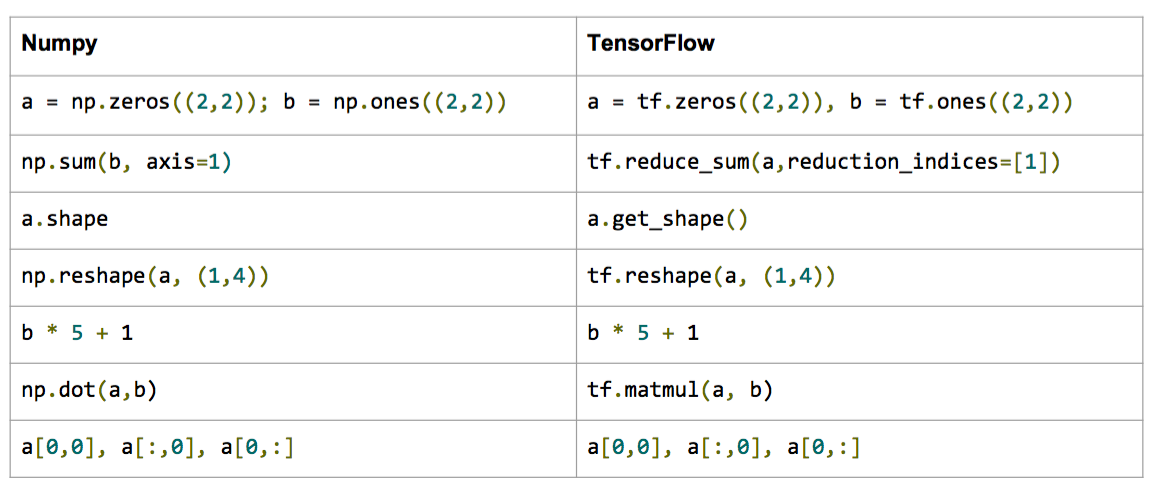Tensorflow的输出要稍微注意一下，我们需要显式地输出(evaluation，也就是说借助eval()函数)！

## 5.Tensorflow的计算图

import tensorflow as tf
# 创建一个常量节点， 产生一个1x2矩阵，这个op被作为一个节点
# 加到默认视图中
# 构造器的返回值代表该常量节点的返回值
matrix1 = tr.constant([[3., 3.]])

# 创建另一个常量节点, 产生一个2x1的矩阵
matrix2 = tr.constant([[2.], [2.]])

# 创建一个矩阵乘法matmul节点，把matrix1和matrix2作为输入：
product = tf.matmul(matrix1, matrix2)## 6.Session对象

Session是用于张量计算的封装环境。具体参考Session文档

# 创建session，启动默认图
# 默认图为tf.Graph().as_default(),一般不用明确写出
# tf.Graph().as_default()
sess = tf.Session()

# 调用sess的'run()' 方法来执行矩阵乘法节点操作，传入'product'作为该方法的参数。'product'代表了矩阵乘法节点的输出，传入它是告诉方法我们希望取回矩阵乘法节点的输出。

#整个执行过程是自动化的，会话负责传递节点所需的全部输入。节点通常是并发执行的。

# 函数调用'run(product)'会触发图中三个节点（上面例子里提到的两个常量节点和一个矩阵乘法节点）的执行。

# 返回值'result'是一个numpy 'ndarray'对象。

result = sess.run(product)
print result
# 结果为[[12.]]

# 完成任务，记得关闭会话
sess.close()


# 用with代码来自动完成session里的图运算并关闭
with tf.Session() as sess:
result = sess.run([product])
print result


# 进入一个交互式Tensorflow会话
import tensorflow as tf
sess = tf.InteractiveSession()

x = tf.Variable([1.0, 2.0])
a = tf.constant([3.0, 3.0]);

# 使用初始化器的run()方法初始化x
x.initializer.run()

# 增加一个减法节点，从x减去a。运行减法op，输出结果
sud = tf.sub(x, a)
print sub.eval()
# 结果为[-2. -1.]


tf.InteractiveSession()是ipython里保持一个默认的session开启的一种便捷的方法。 sess.run(c)其实是一种fetch（获取）操作，它会返回执行c后返回到c的值。后面会说到。

## 7.Session与多GPU运算

Tensorflow是支持分布式的深度学习框架/包，这是因为它能将图定义转换成分布式执行的操作，以充分利用可以利用的计算资源（如CPU或GPU）。不过一般情况下，你不需要显式指定使用CPU还是GPU，Tensorflow能自动检测。如果检测到GPU，Tensorflow会优先使用找到的第一个GPU来执行操作。

# 手动指定给某个gpu执行
with tf.Session() as sess:
with tf.device("/gpu:1"):
matrix1 = tf.constant([[3., 3.]])
matrix2 = tf.constant([[2.], [2.]])
product = tf.matmul(matrix1, matrix2)


• /cpu:0:机器的CPU
• /gpu:0:机器的第一个GPU，如果有的话
• /gpu:1:机器的的第二个GPU，其他GPU以此类推

## 8.Tensorflow的变量张量Variable（常量张量为Constant）

Variable一般是可以被更新或更改的数值，即在流图运行过程中可以被不断动态调整的值。

In : W1 = tf.ones((2,2))
In : W2 = tf.Variable(tf.zeros((2,2)), name="weights")
In : with tf.Session() as sess:
print(sess.run(W1))
sess.run(tf.initialize_all_variables())
print(sess.run(W2))
....:
[[ 1.  1.]
[ 1.  1.]]
[[ 0.  0.]
[ 0. 0.]]


# 用常量初始化变量
In : W = tf.Variable(tf.zeros((2,2)), name="weights")
# 用随机数初始化变量
In : R = tf.Variable(tf.random_normal((2,2)), name="random_weights")
# 最好有with的这种方法打开session，该作用域结束时会自动关闭session
In : with tf.Session() as sess:
....:     sess.run(tf.initialize_all_variables()) # 一定要有！此时才是真正用具体值初始化所有变量
....:     print(sess.run(W))
....:     print(sess.run(R))
....:


In : state = tf.Variable(0, name="counter")
In : new_value = tf.add(state, tf.constant(1))
In : update = tf.assign(state, new_value)
In : with tf.Session() as sess:
sess.run(tf.initialize_all_variables())
print(sess.run(state))
for _ in range(3):
sess.run(update)
print(sess.run(state))
1
2
3


## 9.Fetch（获取）操作

sess.run([node1,node2,...])一般表示我整个流图跑一次，然后显示node1node2……的输出。

input1 = tf.constant(3.0)
input2 = tf.constant(2.0)
input3 = tf.constant(5.0)
mul = tf.mul(input1, intermed)

with tf.Session() as sess:
result = sess.run([mul, intermed])
print result

# print
# 输出最后的乘法结果，和之前的加法结果[27.0, 7.0]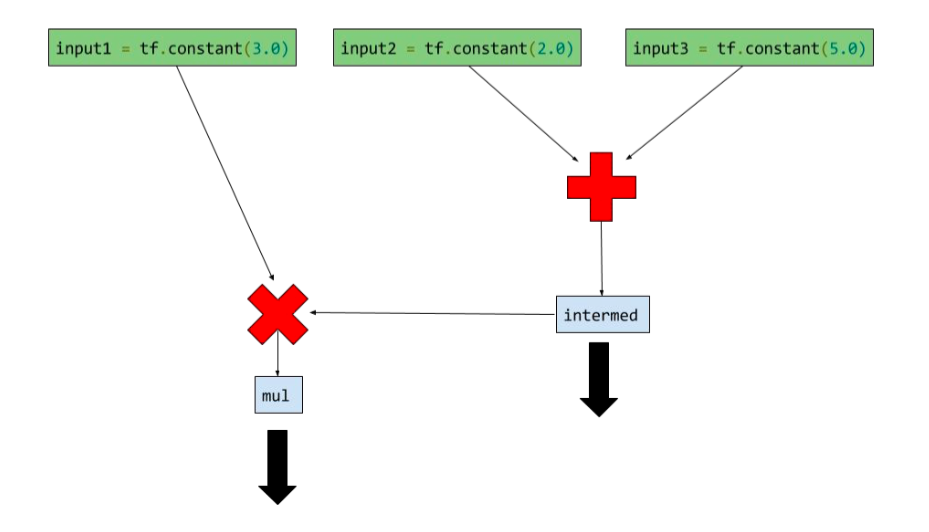In : a = np.zeros((3,3))
In : ta = tf.convert_to_tensor(a)
In : with tf.Session() as sess:
....:     print(sess.run(ta))
....:
[[0. 0. 0.]
[0. 0. 0.]
[0. 0. 0.]]


## 10.Feed（传入）操作

input1 = tf.placeholder(tf.types.float32)	# 输入节点（input op）
input2 = tf.placeholder(tf.types.float32) # 输入节点
output = tf.mul(input1, input2) # 乘法节点，也是一个输出节点


#### 手动提供feed数据作为run的参数

feed_dict是一个python的字典，里面存储从输入变量名称映射到具体的数据。

with tf.Session() as sess:
print sess.run([output], feed_dict={input:[7.]， input2:[2.]})
# print
# 结果是[array([ 14.], dtype=float32)]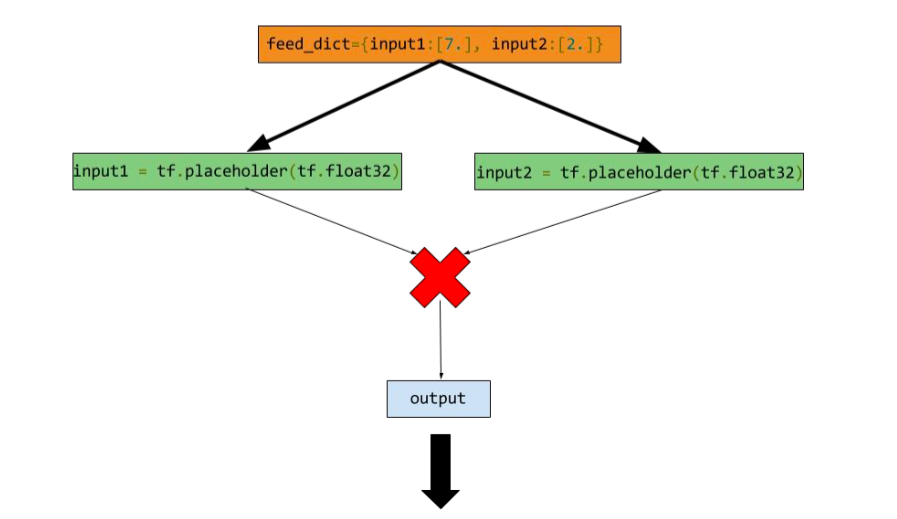## 11.变量范围（Scope）

• tf.variable_scope(scopename)提供了命名空间
• tf.get_variable()在一个命名空间中创建或获取变量
with tf.variable_scope("foo"):
with tf.variable_scope("bar"):
v = tf.get_variable("v", )
assert v.name == "foo/bar/v:0"


with tf.variable_scope("foo"):
v = tf.get_variable("v", )
tf.get_variable_scope().reuse_variables()
v1 = tf.get_variable("v", )
assert v1 == v


#### reuse开关

with tf.variable_scope("foo"):
v = tf.get_variable("v", )
with tf.variable_scope("foo", reuse=True):
v1 = tf.get_variable("v", )
v2 = tf.get_variable("v1",)
assert v1 == v

ValueError: Variable foo/v1 does not exist, or was not created with tf.get_variable(). Did you mean to set reuse=None in VarScope?

with tf.variable_scope("foo"):
v = tf.get_variable("v", )
with tf.variable_scope("foo", reuse=True):
v1 = tf.get_variable("v", )
assert v1 == v

True


reuse默认为False。

def test():
def v1():
with tf.variable_scope("foo") as scope:
for j in range(2):
if j>0:
scope.reuse_variables()
v = tf.get_variable("v", , initializer=tf.constant_initializer(1))

def v2():
with tf.variable_scope("foo", reuse=True) as scope:
for i in range(2):
v = tf.get_variable("v", )

sess = tf.Session()
sess.run(tf.initialize_all_variables())
for i in range(3):
print res, res2

output:
[array([ 2.], dtype=float32)] [array([ 3.], dtype=float32)]
[array([ 4.], dtype=float32)] [array([ 5.], dtype=float32)]
[array([ 6.], dtype=float32)] [array([ 7.], dtype=float32)]


## 12.小例子：使用Tensorflow做线性回归

#### 计算图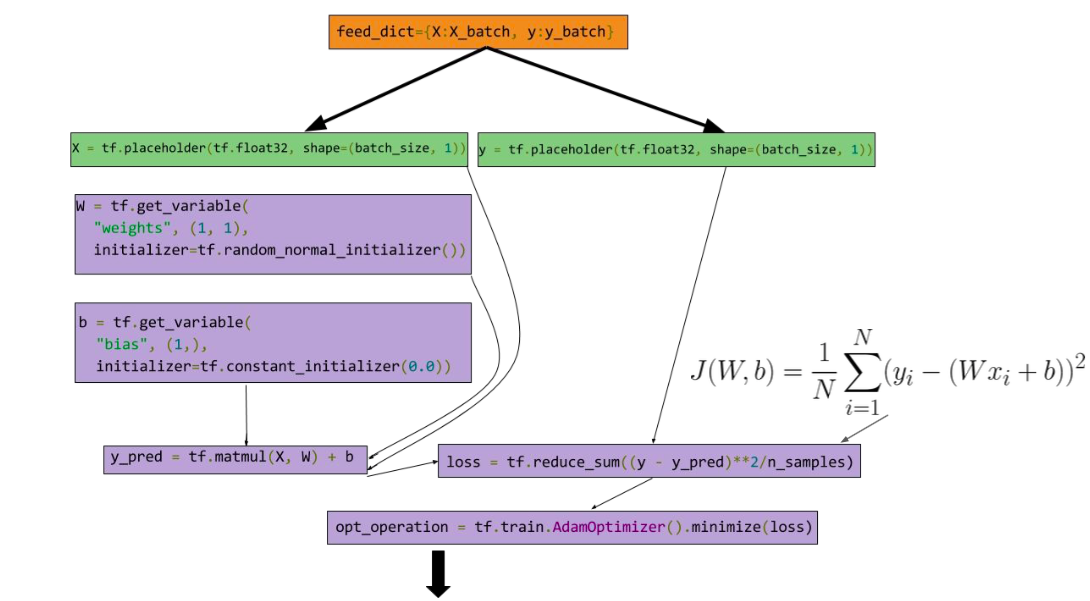#### 代码

import numpy as np
import tensorflow as tf
from matplotlib import pyplot as plt

# 输入的数据（x,y）
X_data = np.arange(100, step=.1)
Y_data = X_data + 20*np.sin(X_data/10)

# 绘制输入的数据
plt.scatter(X_data, Y_data)

# 设置输入数据数量（1000）和SGD的批量数据大小（100）
n_Samples = 1000
batch_size = 100

# 调整数据的shape
X_data = np.reshape(X_data, (n_Samples, 1))
Y_data = np.reshape(Y_data, (n_Samples, 1))

# 设置输入节点
x = tf.placeholder(tf.float32, (batch_size, 1), name='features')
y = tf.placeholder(tf.float32, (batch_size, 1), name='labels')

# 设置变量节点
with tf.variable_scope('Linear-Regression'):
# 权重
w = tf.get_variable(name='weights',shape=(1,1),dtype=tf.float32,initializer=tf.random_normal_initializer())
# bias偏差
b = tf.get_variable(name='bias', shape=(1,),dtype=tf.float32,initializer=tf.constant_initializer(.1))
y_pred = tf.matmul(x,w) + b
# 损失函数
loss = tf.reduce_sum((y_pred-y)**2/batch_size)

# 打开一个session
with tf.Session() as sess:
# 初始化所有变量
sess.run(tf.initialize_all_variables())
# 迭代10000次
for _ in range(1000):
# 数据切片，随机从输入数据中获取batch
indices = np.random.choice(n_Samples, batch_size)
# 特征x
feat = X_data[indices,:]
# 对应的y
lab = Y_data[indices,:]
# feed和fetch
_, l, yp = sess.run([opt_operation, loss, y_pred], feed_dict={x:feat, y:lab})
# 输出每次迭代的损失
print l

# 绘制回归曲线
plt.scatter(feat.flatten(), yp.flatten(), c='r')
# 显示图形
plt.show()


#### 绘制的结果：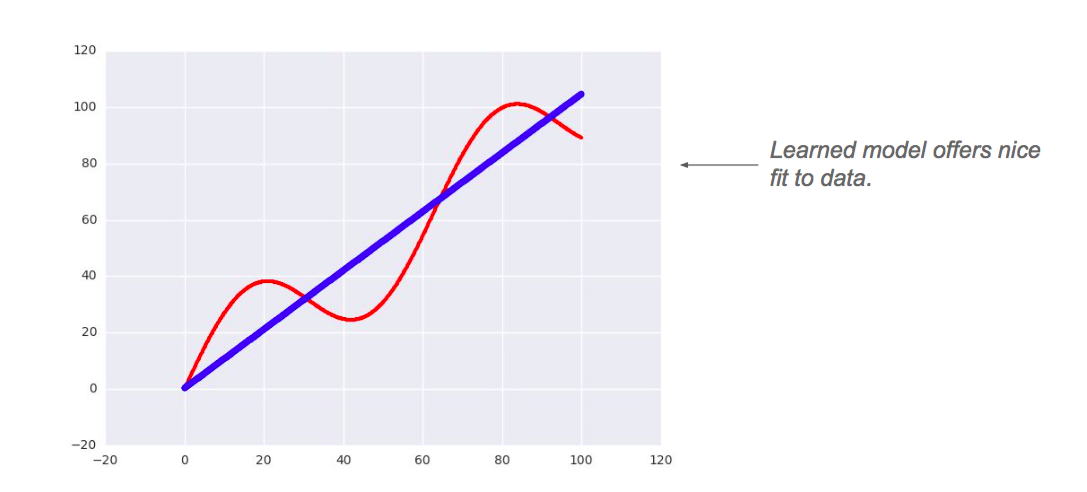1.Tensorflow中的优化函数比较丰富，可以按照实际情况选择。
2.一旦计算图绘制好，我们就可以通过BP求得每个变量节点的偏导数，从而可以更新每个需要更新的变量。而这一切（求导、更新参数）都是通过Tensorflow的优化函数自动完成的。也就是说，我们不用每次求损失函数关于变量（参数）的导数啦！

## 13.TensorBoard

Tensorflow内建的可视化工具。